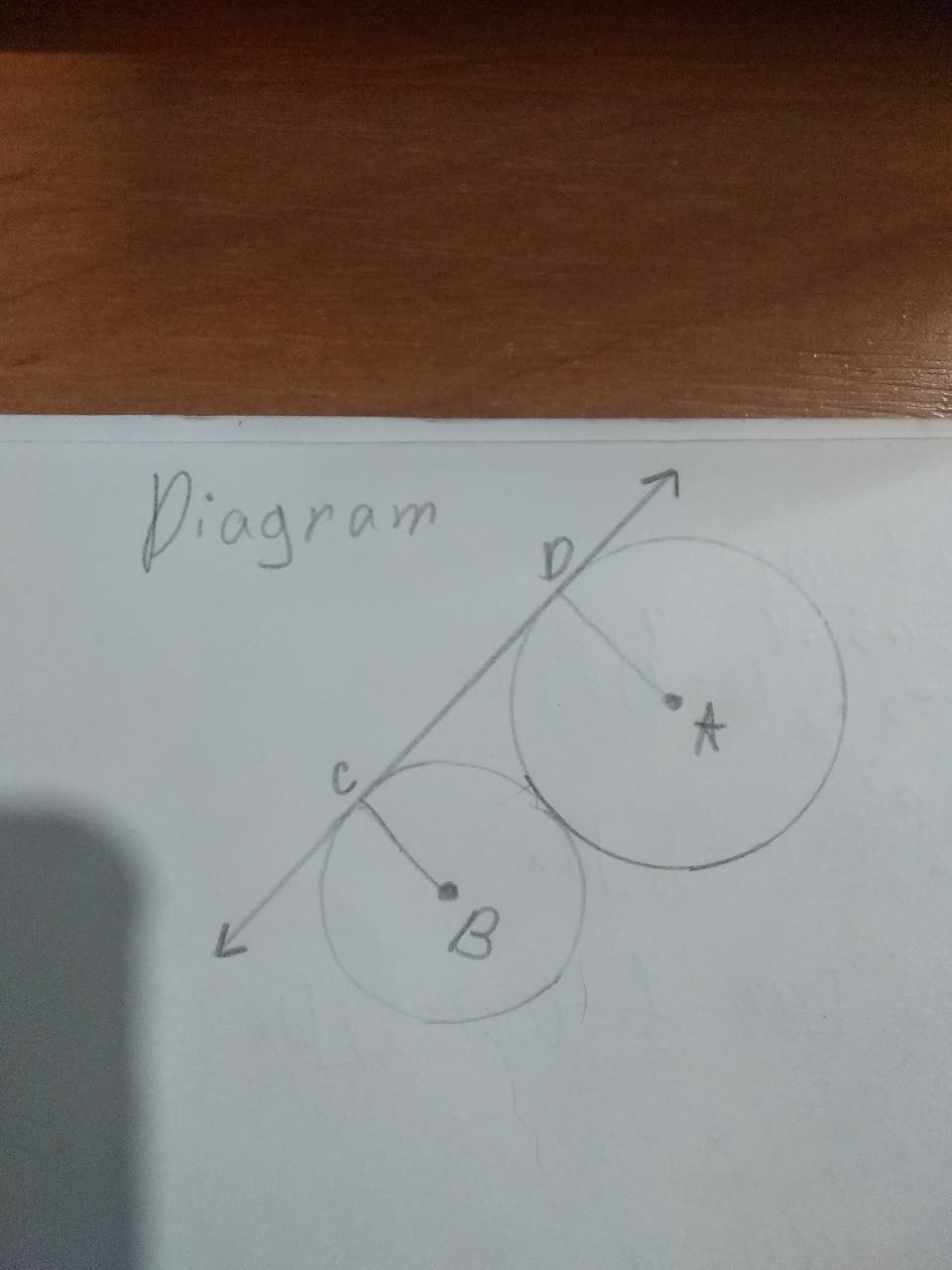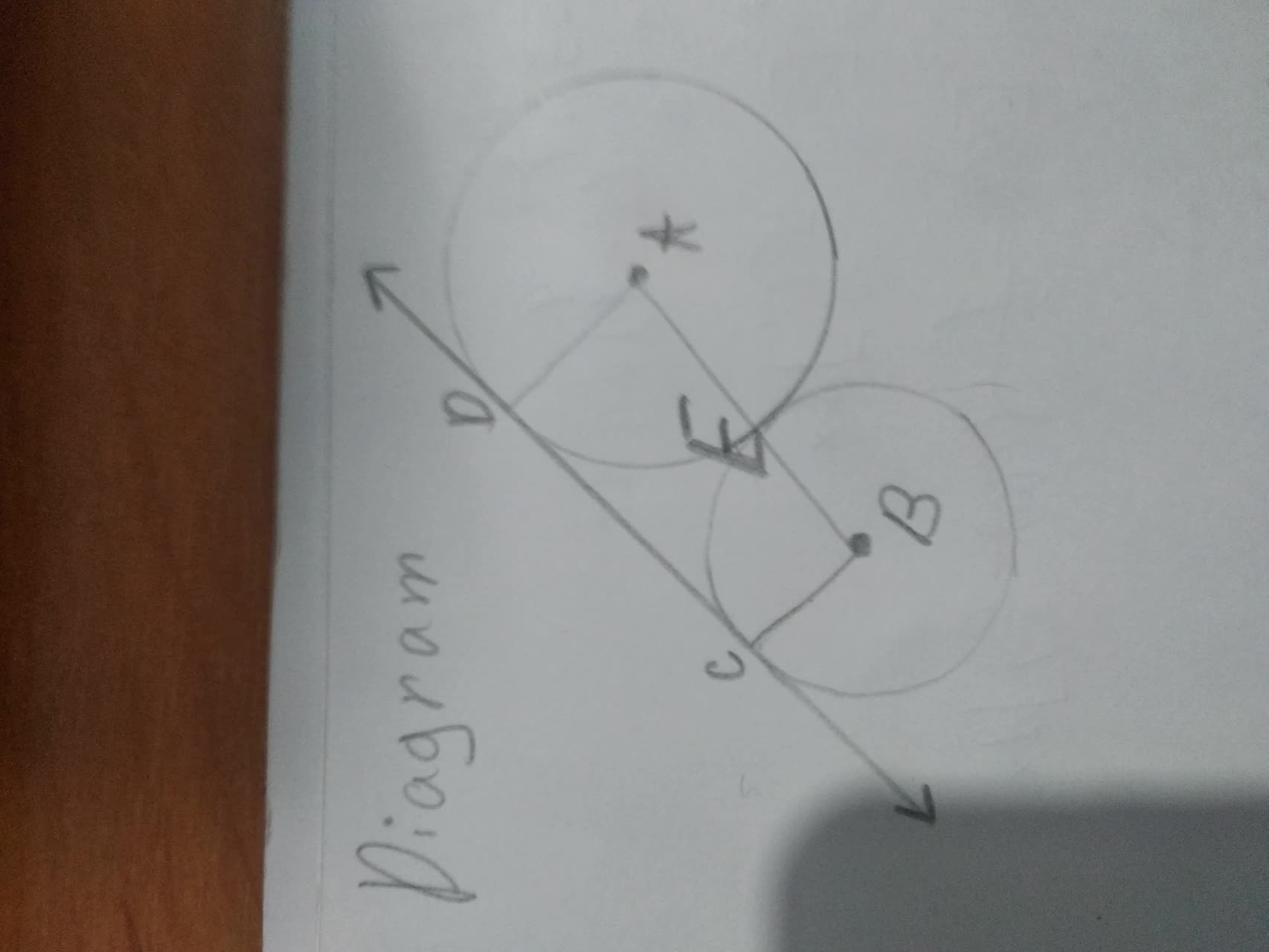# In the diagram, CD is tangent to circles A andjippie771h 2021-11-23 AnsweredIn the diagram, CD is tangent to circles A and B, and he circles are tangent to each other. Answer this question If CD = 15 and AB = 17 and CB = 6, find AD = ?
You can still ask an expert for help

• Questions are typically answered in as fast as 30 minutes

Solve your problem for the price of one coffee

• Math expert for every subject
• Pay only if we can solve itmenerkupvd
Given, In the diagram, CD is tangent to circles A nad B amd the circles are tangent to each other.
CD=15, AB=17 and CB=6
Now,From the diagram, we can say that CB and BE is the radius of the circle B and AD and AE is the radius of the circle A.
So, $\therefore AB=BE+AE\left[\because CB=BE\right]$
$⇒17=CB+AE$
$⇒17=6+AE$
$⇒AE=17-6$
$⇒AE=11$
$\therefore AD=AE=11$
$\therefore AD=11$